Technical Article

# Examining Series RL Circuit Behavior

April 18, 2023 by Amna Ahmad

## The behavior of a series RL circuit can be described using various electrical properties such as resistance, inductance, and impedance. Understanding these properties is essential to analyzing and designing circuits in electrical engineering.

In a series RL circuit, the resistor and the inductor are connected in a series configuration, meaning the current flowing through the circuit passes through both components sequentially. The inductor in the circuit generates a magnetic field that stores energy in the form of an electromagnetic field. The resistor, on the other hand, resists the flow of current and dissipates the energy stored in the circuit.

A series circuit consisting of inductance (L) and resistance (R) is shown in Figure 1(a), and the waveforms and phasor diagram for the circuit are illustrated in Figures 1(b) and (c), respectively. Referring to the circuit diagram, it is seen that (as for all series circuits) the current I is common to both R and L, so the circuit waveforms are drawn starting with the waveform of current.

The voltage (VR) across the resistance is always in phase with the current through the resistance. Thus, the waveform of VR in Figure 1(b) is drawn in phase with the current waveform. The inductor voltage (VL) leads the current through the inductor by 90°. So, the waveform of VL is drawn in Figure 1(b), leading I by 90°. The applied voltage E is the resultant of the two-component voltages, VR and VL, and its waveform can be obtained simply by summing the instantaneous levels of VR and VL. E leads I by an angle (φ) less than 90°.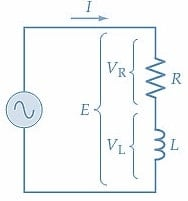##### (a) Series RL circuit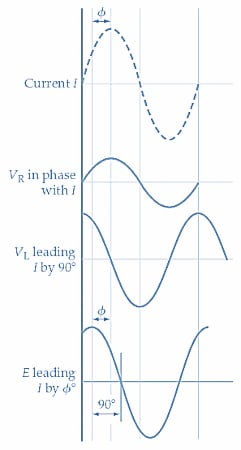##### (b) Circuit waveforms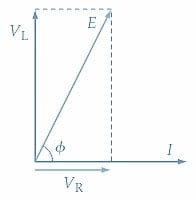### Phasor Diagram

The phasor diagram for the series RL circuit is drawn by starting with the current phasor because the current is the common quantity in a series circuit. A horizontal line is drawn to scale representing current I [ Figure 1(c)]. Because VR is in phase with I, another horizontal line is drawn alongside I to represent VR. The inductor voltage (VL) is 90° ahead of I; so the phasor for VL is drawn vertically at an angle of 90° with respect to I. The phasor addition of VL and VR gives a result that represents the applied voltage (E). Once again, it is seen that the applied voltage leads the circuit current by an angle φ, which is less than the 90° angle that would exist between E and I in a purely inductive circuit.

#### Circuit Equations

E can be expressed in rectangular or polar form. Referring to Figure 1(c)

$E=V_{R}+jV_{L}\,\,\,(1)$

Or in polar form

$E=\sqrt{V^{2}_{R}+V^{2}_{L}}\angle\Big(\frac{V_{L}}{V_{R}}\Big)\,\,\,(2)$

Dividing Equation 1 by I gives

$\frac{E}{I}=\frac{V_{R}}{I}+j\frac{V_{L}}{I}$

The quantity VR/I is the voltage across the resistance divided by the current through the resistance. So, VR/I can be replaced with R. Also, VL/I is the inductor voltage divided by the current through the inductor, which can be replaced with the inductive reactance XL. The quantity E/I is neither resistance nor inductive reactance because it has both component parts. In this case, E/I is termed impedance and is given the symbol Z. Therefore, as illustrated in Figure 2, the above equation can be restated.

Impedance

$Z=R+jX_{L}\,\,\,(3)$

When R and XL are expressed in ohms, the units of Z are also ohms. The numerical value or modulus of Z (i.e., not including the angle) is written as Z.

So

$|Z|=\sqrt{R^{2}+X^{2}_{L}}\,\,\,(4)$

The phase angle of Z is

$\Phi=\Big(\frac{X_{L}}{R}\Big)\,\,\,(5)$

Combining Equations 4 and 5

$E=\sqrt{R^{2}+X^{2}_{L}}\angle\Big(\frac{X_{L}}{R}\Big)\,\,\,(6)$

Equation 6 represents the conversion from rectangular form (Z=R +jX) to polar form (Z∠ϕ).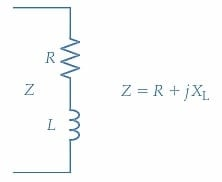#### Impedance Diagram

The quantities Z, R, and XL can be represented on a vector diagram. They are not phasor quantities because they have fixed values. Unlike ac voltage and current quantities, Z, R, and XL do not have continuously changing instantaneous values. To distinguish the vector diagram of impedance, reactance, and resistance from a phasor diagram, it is usually drawn in triangular form and is referred to as an impedance diagram. Figure 3 shows the impedance diagram for the series RL circuit of Figure 1(a). A horizontal line is first drawn to represent the resistive component R. The +j component (XL) is then drawn at +90° with respect to R, as illustrated. The Z component is the hypotenuse of the triangle, and the angle φ is the phase angle of the impedance Z with respect to resistance R.##### Figure 3. Impedance diagram (or impedance triangle) for a series RL circuit. Inductive reactance vector XL is drawn (up) from the resistance vector at an angle of 90°. The impedance (Z∠ɸ) is the result of R and XL. Image used courtesy of Amna Ahmad

As in the case of conductance (the reciprocal of resistance) and susceptance (the reciprocal of reactance), the reciprocal of impedance is an important quantity that can be usefully applied in circuit analysis. The admittance (symbol Y) is the reciprocal of impedance (Z), and its unit is the siemens (S).

$Y=\frac{1}{Z}\,\,\,(7)$

When Z is expressed in ohms in Equation 7, Y is in siemens.

### Practical Inductors

All inductors are coils with some winding resistance, and as already explained, practical inductors can be represented by a pure inductance in series with the winding resistance. So, the diagrams in Figures 1 and 3 can apply to the case of an inductor connected directly to an ac supply. Where an external resistance is connected in series with the inductor, the winding resistance should also be shown as a series component in the equivalent circuit.

### Analysis of a Series RL Circuit

Calculate the inductive reactance XL = 2πfL

If there is more than one resistive component, calculate the total resistance:
R = R1 + R2 + …

Calculate the circuit impedance
$|Z|=\sqrt{R^{2}+X^{2}_{L}}$

Calculate the phase angle φ = XLR

Calculate the current I = E/Z

Determine the resistive voltage VR = IR or VR = E cos ɸ

Determine the inductive voltage VL = IXL or VL = E sin ɸ

Example 1

Analyze the series RL circuit in Figure 4 to determine the current, the voltage across R, the voltage across L, and the phase angle of the current with respect to the supply voltage.

Solution

Inductor reactance

$X_{L}=2\pi fL=2\pi\times100Hz\times20mH\approx12.5\Omega$

Circuit impedance

$|Z|=\sqrt{R^{2}+X^{2}_{L}}=\sqrt{(20\Omega)^{2}+(12.57\Omega)^{2}=23.6\Omega}$

Phase angle

$\Phi=\Big(\frac{X_{L}}{R}\Big)=\Big(\frac{12.56\Omega}{20\Omega}\Big)=32.1\degree$

Circuit current

$I=\frac{E}{Z}=\frac{60V}{23.6\Omega\angle32.1\degree}\approx2.54A\angle-32.1\degree$

Referring to the series RL circuit phasor diagram Figure 1(c):

Resistor voltage

$V_{R}=Ecos\Phi=60Vcos32.1\degree=50.8V(=I\times R)$

Inductor voltage

$V_{L}=Esin\Phi=60Vcos32.1\degree=31.9V(=I\times X_{L})$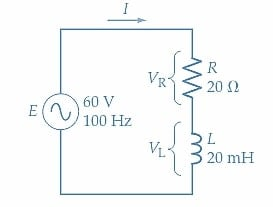### Takeaways of Series RL Circuit Behavior

A series RL circuit is an important electrical circuit that consists of a resistor and an inductor connected in series with a power source. The behavior of a series RL circuit can be described using impedance and phasor diagrams, which provide a graphical representation of the complex impedance and phase relationship between the voltage and current. The impedance diagram represents the impedance of the circuit as a vector in a complex plane, while the phasor diagram represents the phase relationship between the voltage and current as a rotating vector. Understanding these diagrams is essential for analyzing and designing series RL circuits, as well as for understanding the behavior of reactive components in electrical circuits.

Featured image used courtesy of Adobe Stock